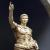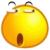## Postgresql Bitstring index for multicolumns

0#### 引用来自“mark35”的答案

POSTGRES Never disappoint you

0#### 引用来自“mark35”的答案

POSTGRES Never disappoint you

0#### 引用来自“mark35”的答案

POSTGRES Never disappoint you

int4[]这种数组情况,你索引如何建立.

0#### 引用来自“mark35”的答案

POSTGRES Never disappoint you

int4[]这种数组情况,你索引如何建立.

030W记录，typeids 创建了普通btree索引，已ANALYZE。

```explain analyze select * from cdb_threads where typeids = ARRAY[2,55]
Index Scan using "threads.typeids" on cdb_threads  (cost=0.00..8.83 rows=2 width=222) (actual time=0.034..0.036 rows=1 loops=1)
Index Cond: (typeids = '{2,55}'::integer[])
Total runtime: 0.076 ms```

```explain analyze select * from cdb_threads where typeids @> ARRAY[2,55]

Seq Scan on cdb_threads  (cost=0.00..57154.50 rows=312 width=222) (actual time=250.078..297.224 rows=1 loops=1)
Filter: (typeids @> '{2,55}'::integer[])
Total runtime: 297.267 ms```0#### 引用来自“mark35”的答案

30W记录，typeids 创建了普通btree索引，已ANALYZE。

```explain analyze select * from cdb_threads where typeids = ARRAY[2,55]
Index Scan using "threads.typeids" on cdb_threads  (cost=0.00..8.83 rows=2 width=222) (actual time=0.034..0.036 rows=1 loops=1)
Index Cond: (typeids = '{2,55}'::integer[])
Total runtime: 0.076 ms```

```explain analyze select * from cdb_threads where typeids @> ARRAY[2,55]

Seq Scan on cdb_threads  (cost=0.00..57154.50 rows=312 width=222) (actual time=250.078..297.224 rows=1 loops=1)
Filter: (typeids @> '{2,55}'::integer[])
Total runtime: 297.267 ms```

0#### 引用来自“mark35”的答案

30W记录，typeids 创建了普通btree索引，已ANALYZE。

```explain analyze select * from cdb_threads where typeids = ARRAY[2,55]
Index Scan using "threads.typeids" on cdb_threads  (cost=0.00..8.83 rows=2 width=222) (actual time=0.034..0.036 rows=1 loops=1)
Index Cond: (typeids = '{2,55}'::integer[])
Total runtime: 0.076 ms```

```explain analyze select * from cdb_threads where typeids @> ARRAY[2,55]

Seq Scan on cdb_threads  (cost=0.00..57154.50 rows=312 width=222) (actual time=250.078..297.224 rows=1 loops=1)
Filter: (typeids @> '{2,55}'::integer[])
Total runtime: 297.267 ms```